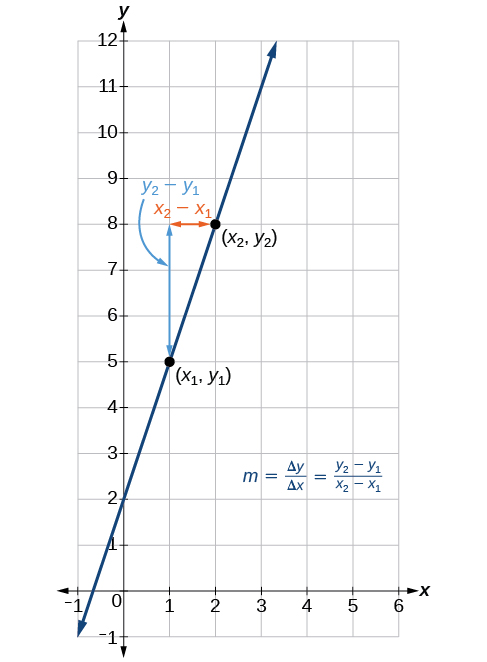# 4.1 Linear functions  (Page 3/27)

 Page 3 / 27

## Increasing and decreasing functions

The slope determines if the function is an increasing linear function    , a decreasing linear function    , or a constant function.

• $f\left(x\right)=mx+b\text{\hspace{0.17em}}$ is an increasing function if $\text{\hspace{0.17em}}m>0.$
• $f\left(x\right)=mx+b\text{\hspace{0.17em}}$ is a decreasing function if $\text{\hspace{0.17em}}m<0.$
• $f\left(x\right)=mx+b\text{\hspace{0.17em}}$ is a constant function if $\text{\hspace{0.17em}}m=0.$

## Deciding whether a function is increasing, decreasing, or constant

Some recent studies suggest that a teenager sends an average of 60 texts per day http://www.cbsnews.com/8301-501465_162-57400228-501465/teens-are-sending-60-texts-a-day-study-says/ . For each of the following scenarios, find the linear function that describes the relationship between the input value and the output value. Then, determine whether the graph of the function is increasing, decreasing, or constant.

1. The total number of texts a teen sends is considered a function of time in days. The input is the number of days, and output is the total number of texts sent.
2. A teen has a limit of 500 texts per month in his or her data plan. The input is the number of days, and output is the total number of texts remaining for the month.
3. A teen has an unlimited number of texts in his or her data plan for a cost of \$50 per month. The input is the number of days, and output is the total cost of texting each month.

Analyze each function.

1. The function can be represented as $\text{\hspace{0.17em}}f\left(x\right)=60x\text{\hspace{0.17em}}$ where $\text{\hspace{0.17em}}x\text{\hspace{0.17em}}$ is the number of days. The slope, 60, is positive so the function is increasing. This makes sense because the total number of texts increases with each day.
2. The function can be represented as $\text{\hspace{0.17em}}f\left(x\right)=500-60x\text{\hspace{0.17em}}$ where $\text{\hspace{0.17em}}x$ is the number of days. In this case, the slope is negative so the function is decreasing. This makes sense because the number of texts remaining decreases each day and this function represents the number of texts remaining in the data plan after $\text{\hspace{0.17em}}x\text{\hspace{0.17em}}$ days.
3. The cost function can be represented as $\text{\hspace{0.17em}}f\left(x\right)=50\text{\hspace{0.17em}}$ because the number of days does not affect the total cost. The slope is 0 so the function is constant.

## Interpreting slope as a rate of change

In the examples we have seen so far, the slope was provided to us. However, we often need to calculate the slope given input and output values. Recall that given two values for the input, $\text{\hspace{0.17em}}{x}_{1}$ and $\text{\hspace{0.17em}}{x}_{2},$ and two corresponding values for the output, $\text{\hspace{0.17em}}{y}_{1}\text{\hspace{0.17em}}$ and $\text{\hspace{0.17em}}{y}_{2}\text{\hspace{0.17em}}$ —which can be represented by a set of points, and —we can calculate the slope $m.$

Note that in function notation we can obtain two corresponding values for the output $\text{\hspace{0.17em}}{y}_{1}\text{\hspace{0.17em}}$ and $\text{\hspace{0.17em}}{y}_{2}\text{\hspace{0.17em}}$ for the function $\text{\hspace{0.17em}}f,$ $\text{\hspace{0.17em}}{y}_{1}=f\left({x}_{1}\right)\text{\hspace{0.17em}}$ and $\text{\hspace{0.17em}}{y}_{2}=f\left({x}_{2}\right),$ so we could equivalently write

$m=\frac{f\left({x}_{2}\right)–f\left({x}_{1}\right)}{{x}_{2}–{x}_{1}}$

[link] indicates how the slope of the line between the points, $\text{\hspace{0.17em}}\left({x}_{1},{y}_{1}\right)\text{\hspace{0.17em}}$ and $\text{\hspace{0.17em}}\left({x}_{2},{y}_{2}\right),\text{\hspace{0.17em}}$ is calculated. Recall that the slope measures steepness, or slant. The greater the absolute value of the slope, the steeper the slant is.The slope of a function is calculated by the change in   y   divided by the change in   x .   It does not matter which coordinate is used as the   ( x 2 , y 2 )   and which is the   ( x 1 , y 1 ) ,   as long as each calculation is started with the elements from the same coordinate pair.

#### Questions & Answers

write down the polynomial function with root 1/3,2,-3 with solution
if A and B are subspaces of V prove that (A+B)/B=A/(A-B)
write down the value of each of the following in surd form a)cos(-65°) b)sin(-180°)c)tan(225°)d)tan(135°)
Prove that (sinA/1-cosA - 1-cosA/sinA) (cosA/1-sinA - 1-sinA/cosA) = 4
what is the answer to dividing negative index
In a triangle ABC prove that. (b+c)cosA+(c+a)cosB+(a+b)cisC=a+b+c.
give me the waec 2019 questions
the polar co-ordinate of the point (-1, -1)
prove the identites sin x ( 1+ tan x )+ cos x ( 1+ cot x )= sec x + cosec x
tanh`(x-iy) =A+iB, find A and B
B=Ai-itan(hx-hiy)
Rukmini
what is the addition of 101011 with 101010
If those numbers are binary, it's 1010101. If they are base 10, it's 202021.
Jack
extra power 4 minus 5 x cube + 7 x square minus 5 x + 1 equal to zero
the gradient function of a curve is 2x+4 and the curve passes through point (1,4) find the equation of the curve
1+cos²A/cos²A=2cosec²A-1
test for convergence the series 1+x/2+2!/9x3ByByByBy MldelatteBy RhodesBy